# Colours and Shapes

Before you get started, read the page on the basics of plotting with ggplot and install the package ggplot2.

library(ggplot2)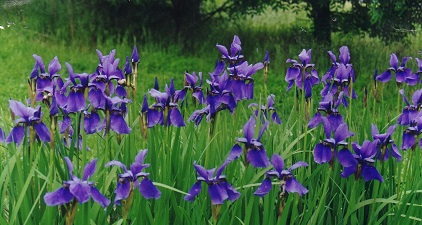In these examples, let’s use a data set that is already in R with the length and width of floral parts for three species of iris. First, load the data set:

data(iris)

The following plots will be used as the base code throughout of this tutorial
* a scatter plot of petal length vs sepal length
* a box plot of sepal length vs species
* a frequency histogram of sepal length

IrisPlot <- ggplot(iris, aes(Sepal.Length, Petal.Length)) +
geom_point()

IrisBox <- ggplot(iris, aes(Species, Sepal.Length, fill = Species)) +
geom_boxplot()

IrisHist <- ggplot(iris, aes(Sepal.Length)) +
geom_histogram()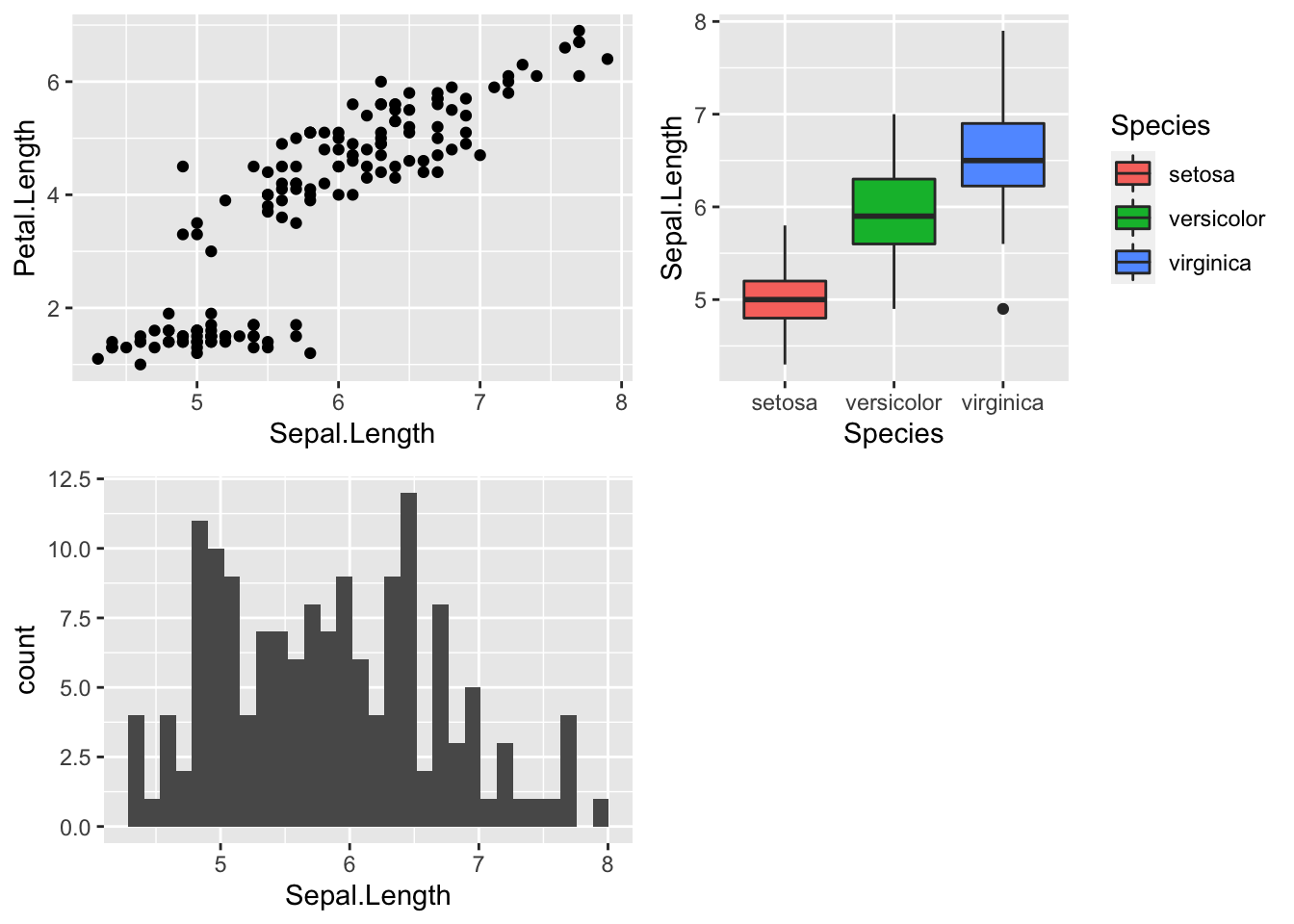### Changing the colour of the whole plot or its outline

To colour your entire plot one colour, add fill = "colour" or colour = "colour" into the brackets following the geom_... code where you specified what type of graph you want.

Note that for most plots, fill = "colour" will colour the whole shape, whereas colour = "colour" will fill in the outline.

For example, to make a blue box plot with a red outline, or a yellow histogram with an green outline::

IrisBox <- ggplot(iris, aes(Species, Sepal.Length)) +
geom_boxplot(fill = "blue", colour = "red")

IrisHist <- ggplot(iris, aes(Sepal.Length)) +
geom_histogram(fill = "yellow", colour = "green")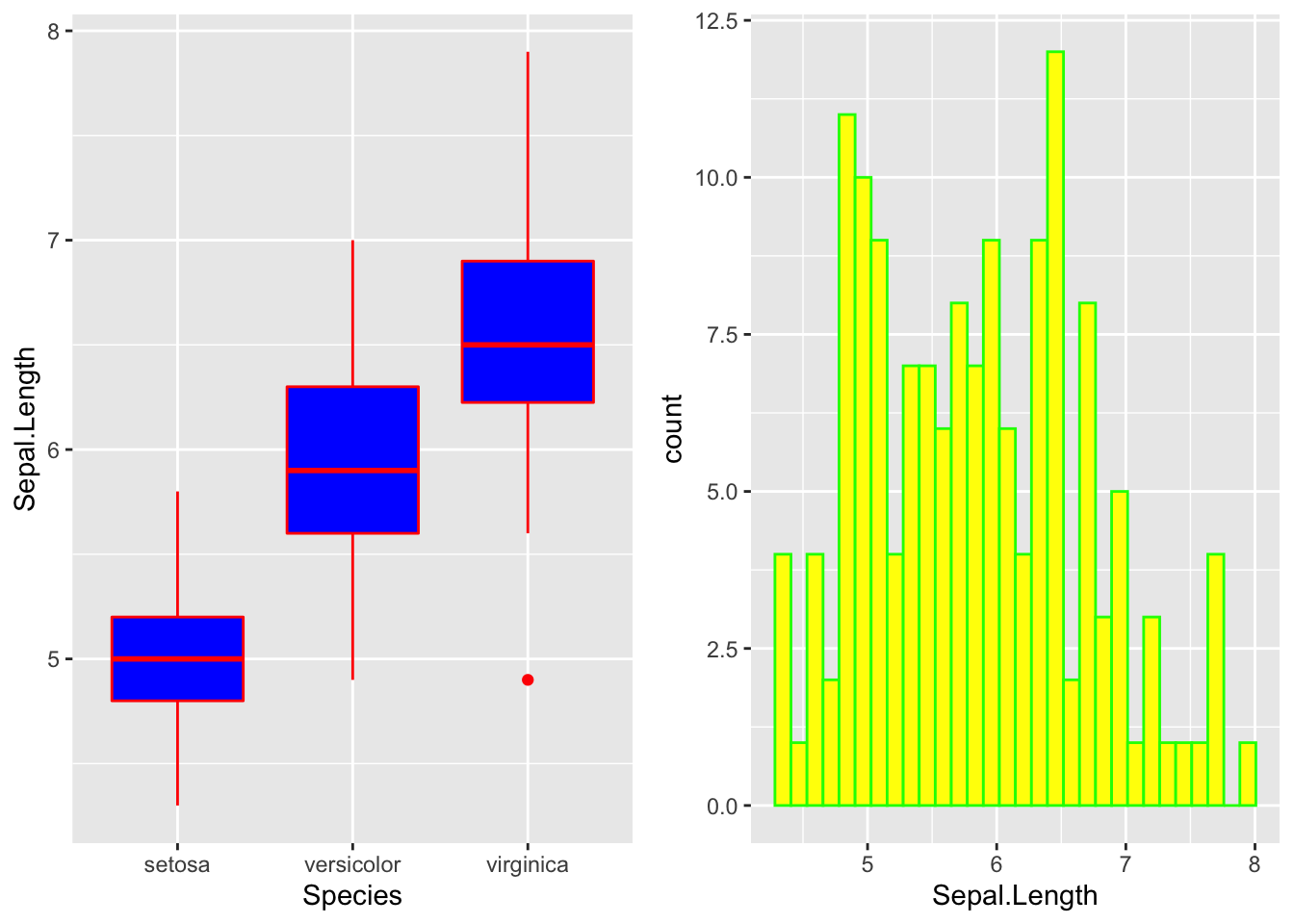For scatterplots, colour = "colour" will specify the fill colour for the point shape.

IrisPlot <- ggplot(iris, aes(Petal.Length, Sepal.Length)) +
geom_point(colour = "red")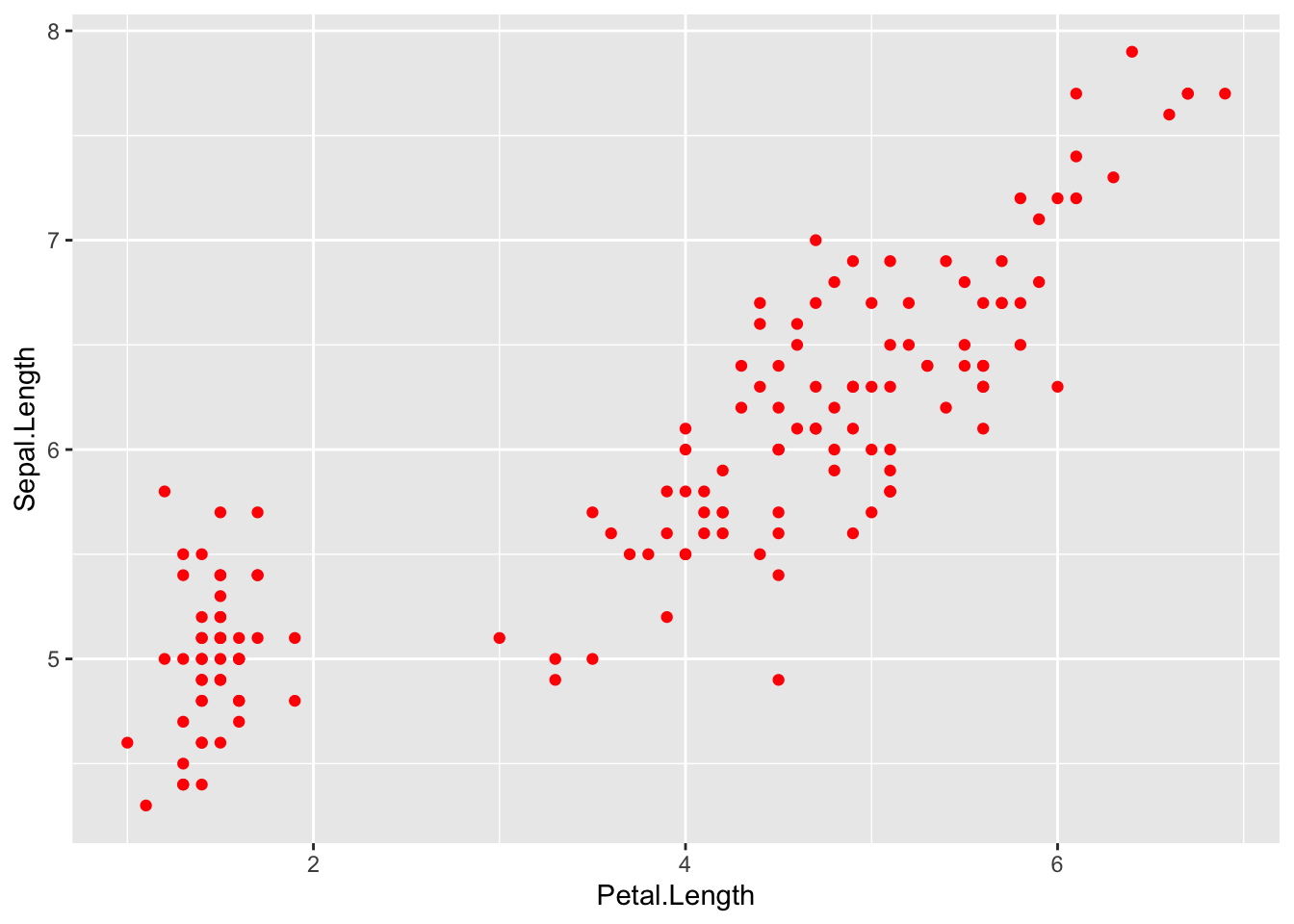The plots and outlines can be changed to any colour listed here. Remember to include “” before and after the colour name.

### Using colour to visualise additional variables

If you wish to colour point on a scatter plot by a third categorical variable, then add colour = variable.name within your aes brackets. This tells ggplot that this third variable will colour the points. To colour the points by the variable Species:

IrisPlot <- ggplot(iris, aes(Petal.Length, Sepal.Length, colour = Species)) +
geom_point()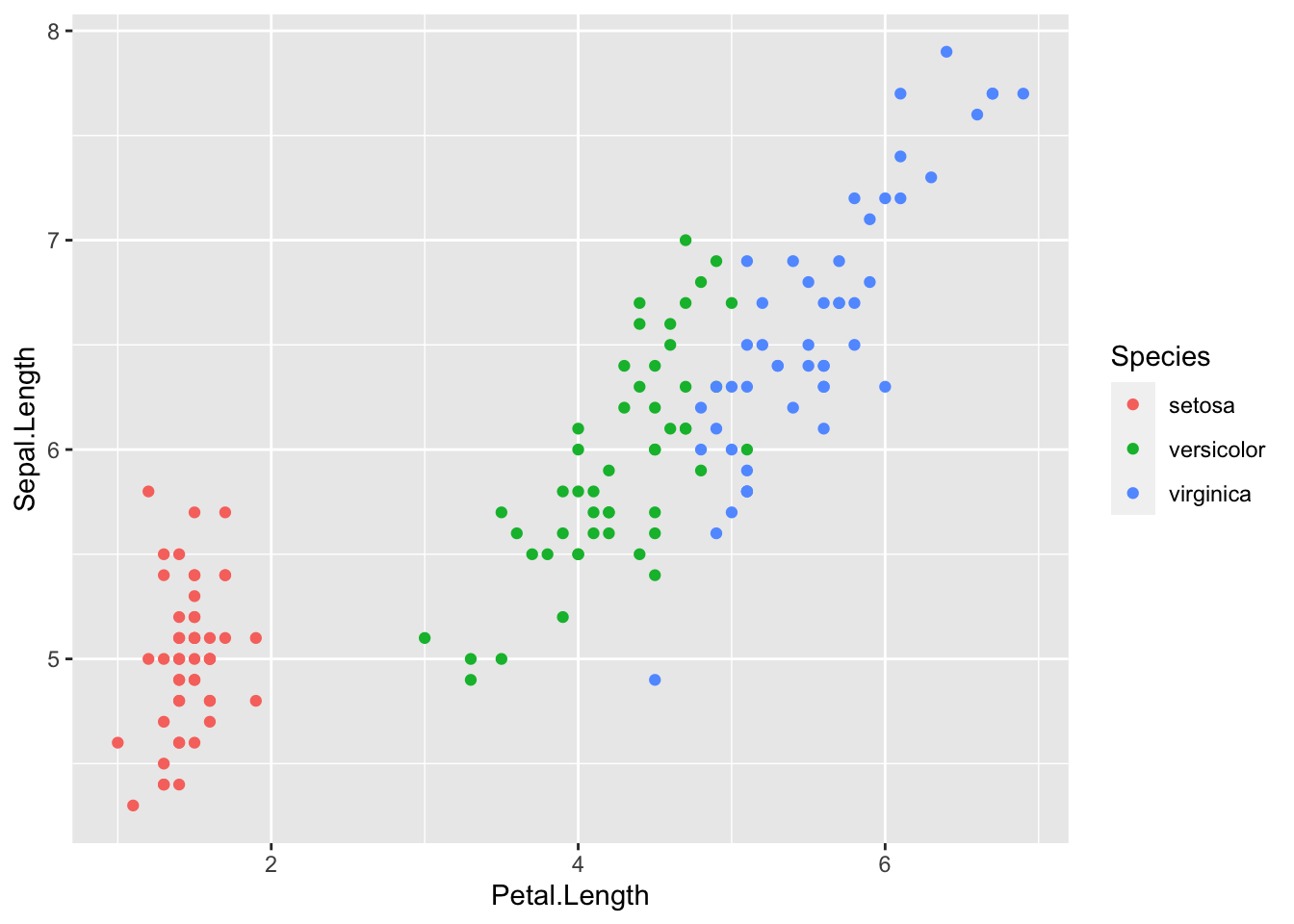To colour box plots or bar plots by a given categorical variable, you use you use fill = variable.name instead of colour.

IrisBox <- ggplot(iris, aes(Species, Sepal.Length, fill = Species)) +
geom_boxplot()The basic format for colouring a continuous variable is very similar to a categorical variable. The only real difference is you need to use + scale_colour_gradient(low = "colour1", high = "colour2"). The other colour scales will not work as they are for categorical variables. For example, here is a plot of sepal length vs petal length, with the symbols colored by their value of sepal width.

IrisPlot.continuous <- ggplot(iris, aes(Petal.Length, Sepal.Length, colour = Sepal.Width)) +
geom_point()

print(IrisPlot.continuous)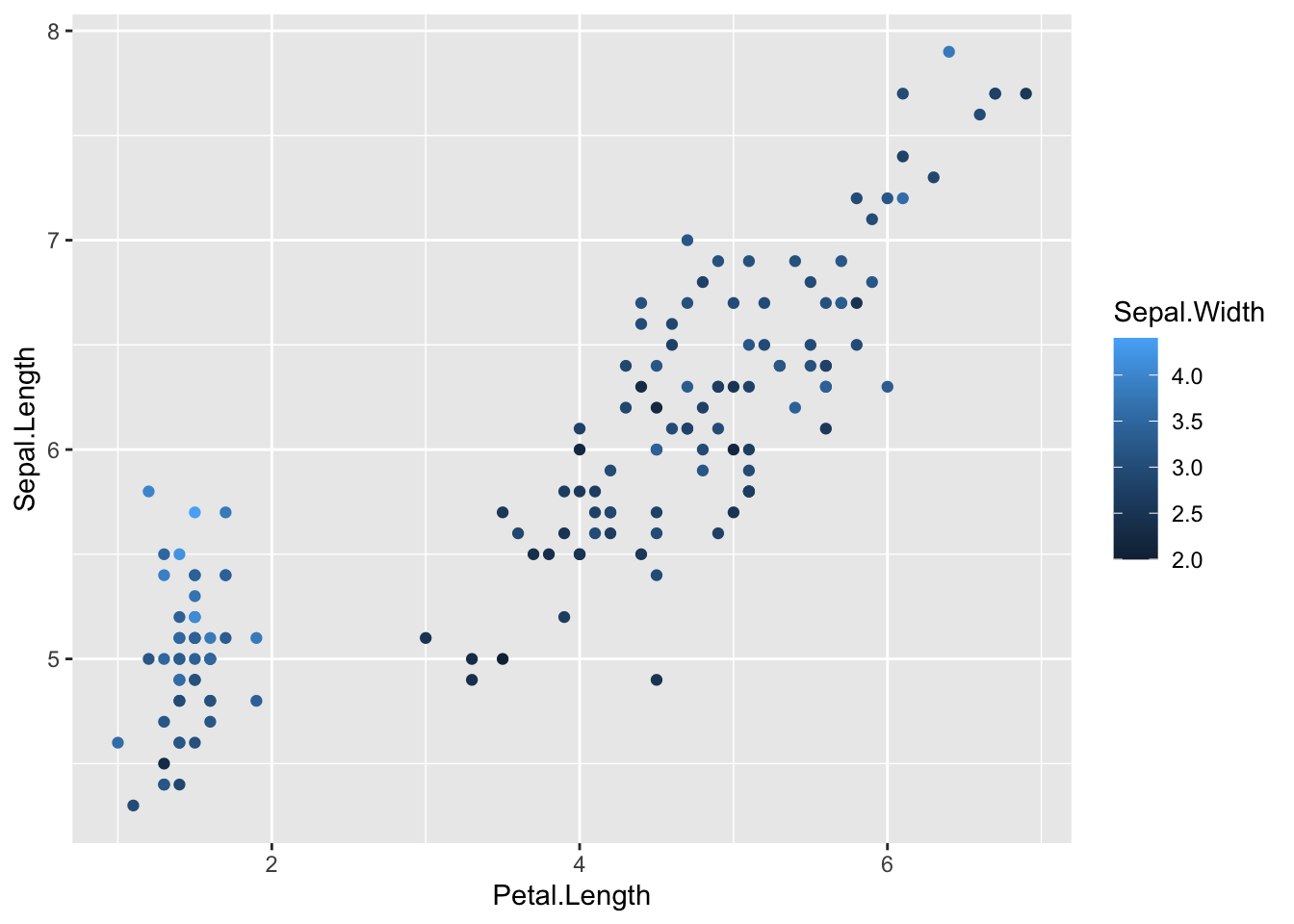To make the gradient more effective, specify two colours within the + scale_colour_gradient brackets to represent either end of the gradient. For example:

print(IrisPlot.continuous + scale_colour_gradient(low = "black", high = "white"))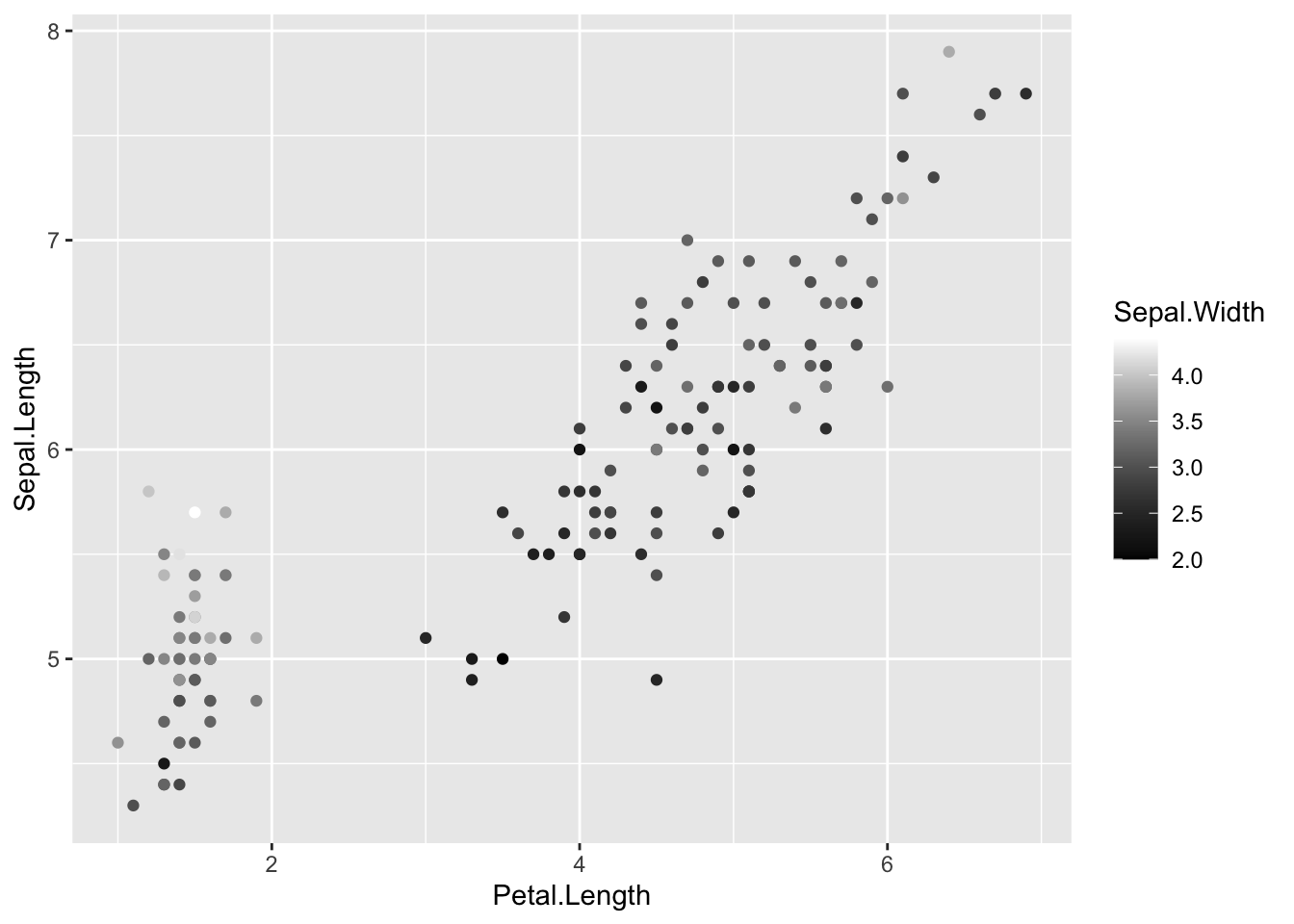print(IrisPlot.continuous + scale_colour_gradient(low = "darkolivegreen1", high = "darkolivegreen"))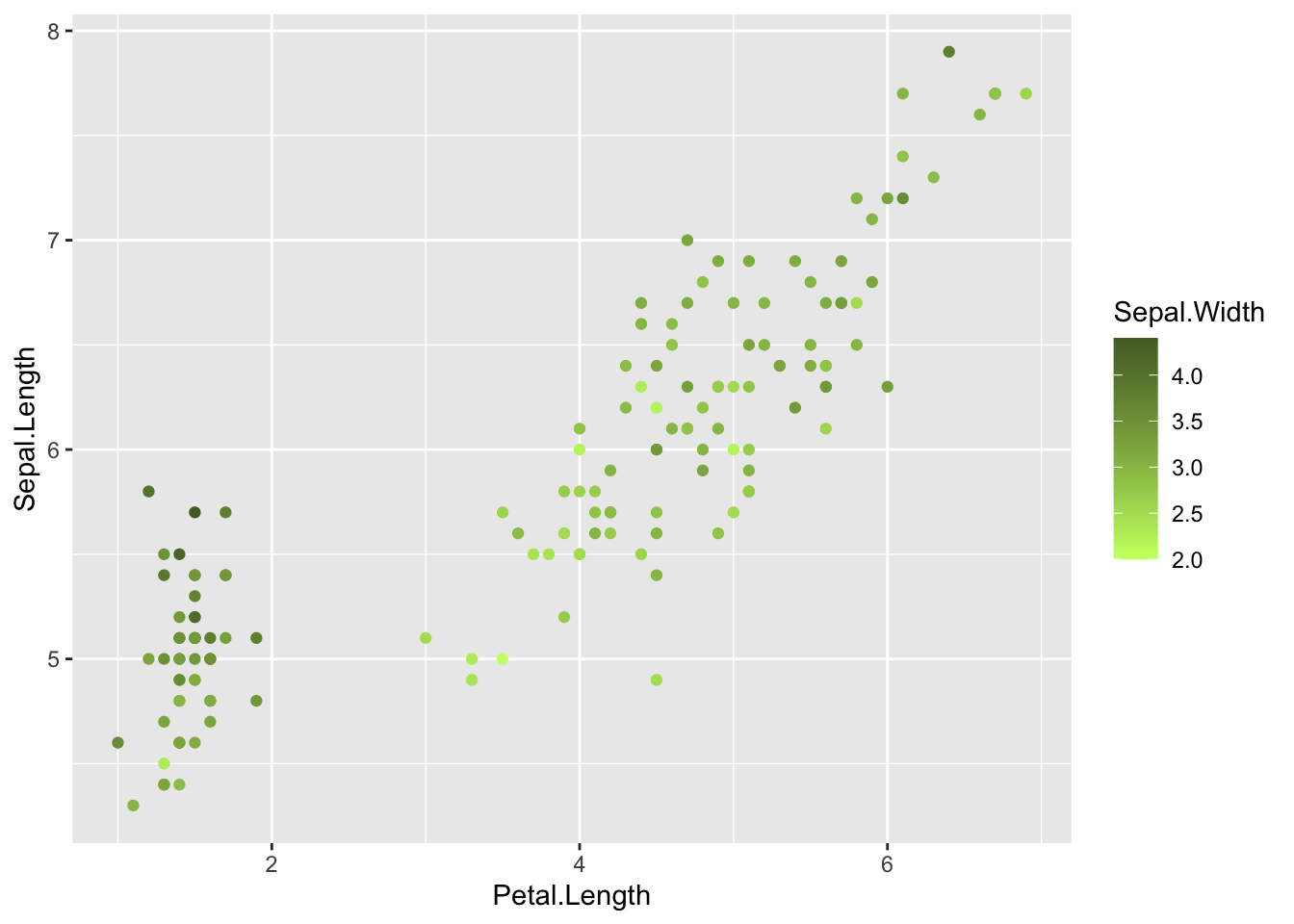### Choosing your own colours for these variables

This can be done in numerous ways. The basic format is to add + scale_colour_yourchoice() for scatter plots or + scale_fill_yourchoice() for box plots to the code where you ‘print’ your graph, where yourchoice() is one of several options. The syntax is:

print(your.basic.graph + your.theme + scale_colour_yourchoice())

There are numerous options for the + scale_colour_yourchoice() part.

Individually select colours. To manually choose colours, you can use + scale_colour_manual() or + scale_fill_manual(). For example, to choose three colours for the iris plots:

print(IrisPlot + scale_colour_manual(values = c("Blue", "Red", "Green")))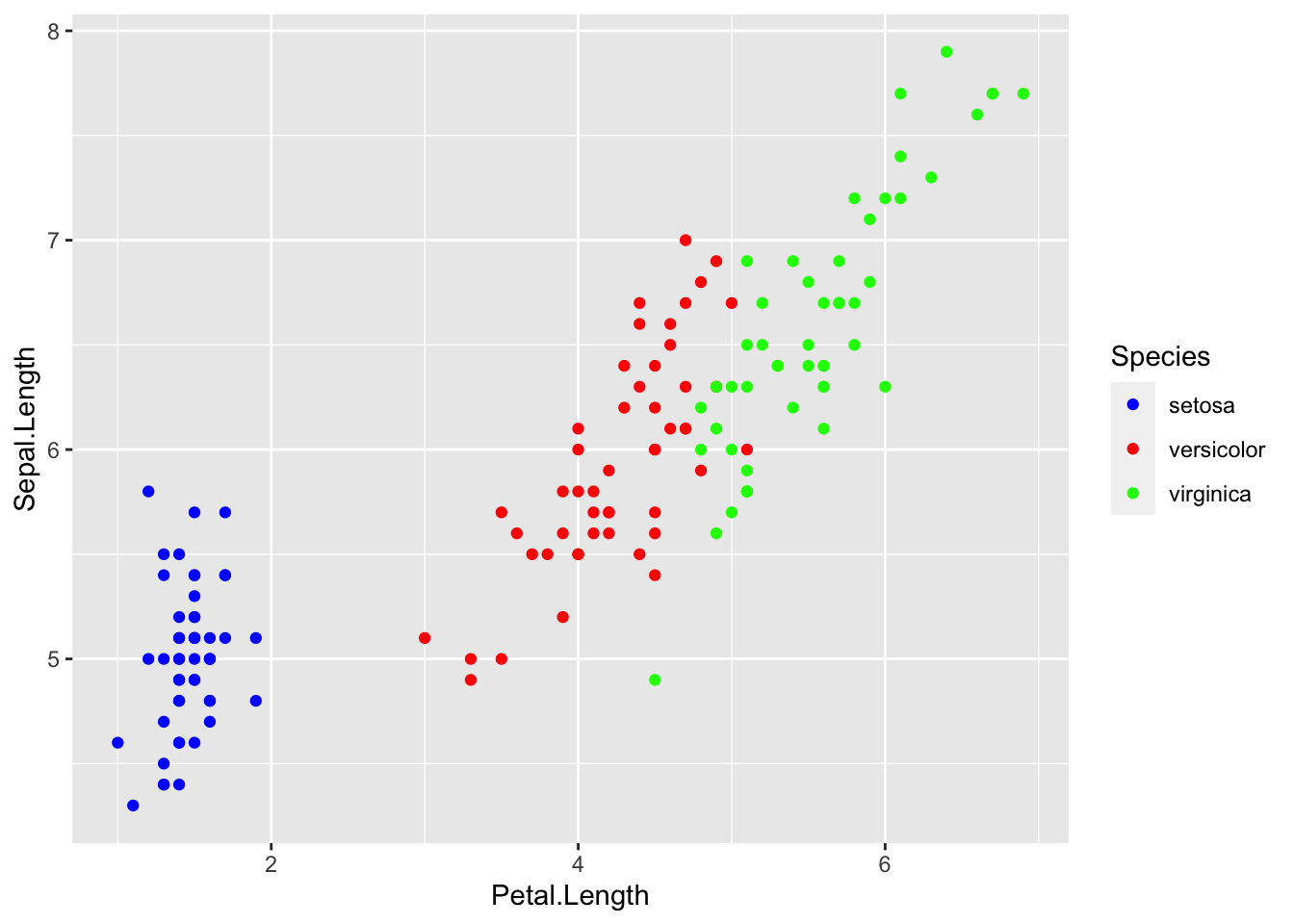print(IrisBox + scale_fill_manual(values = c("Black", "Orange", "Brown")))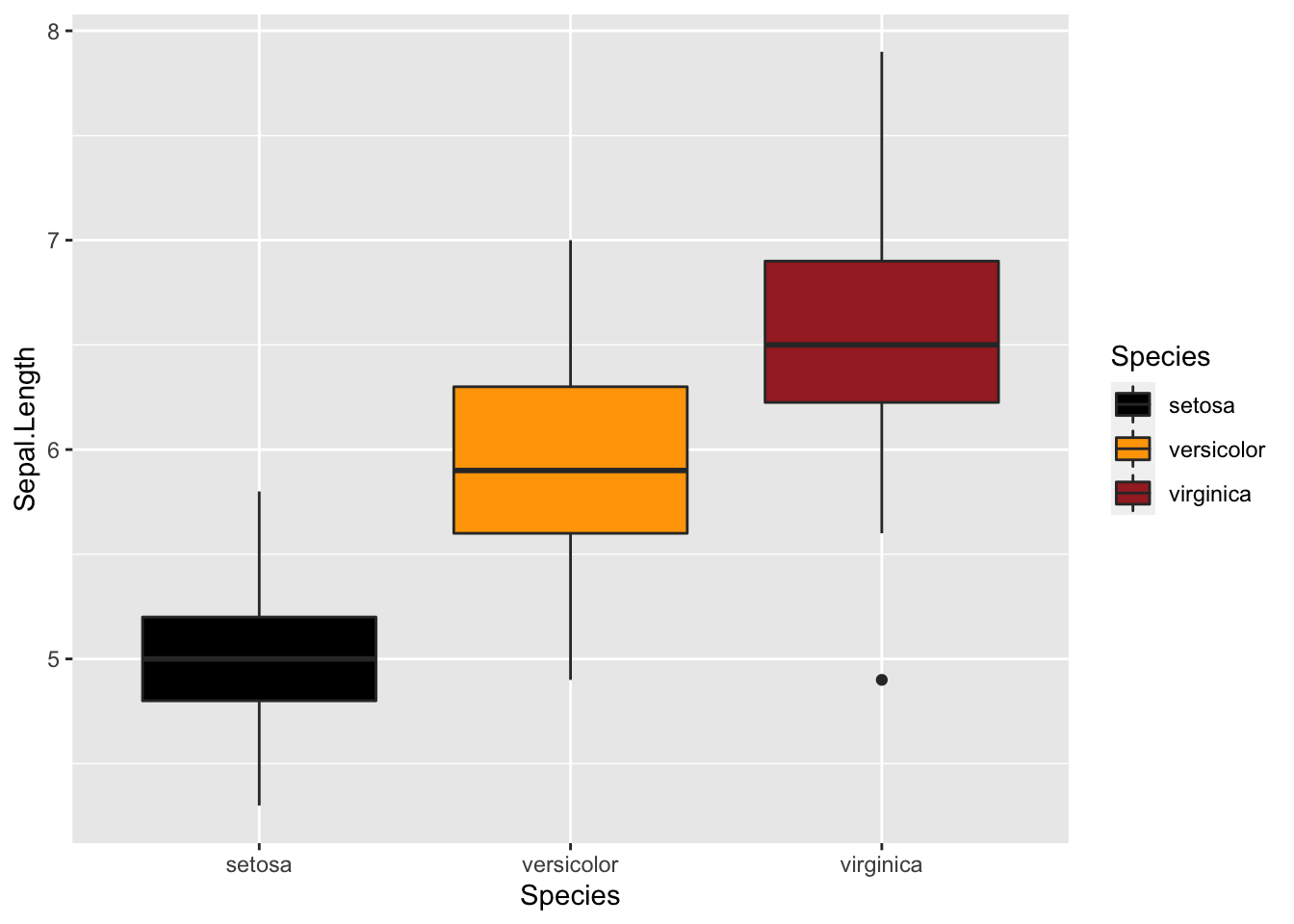Assign tones on a greyscale. Use + scale_colour_grey() or + scale_fill_grey()

print(IrisPlot + scale_colour_grey())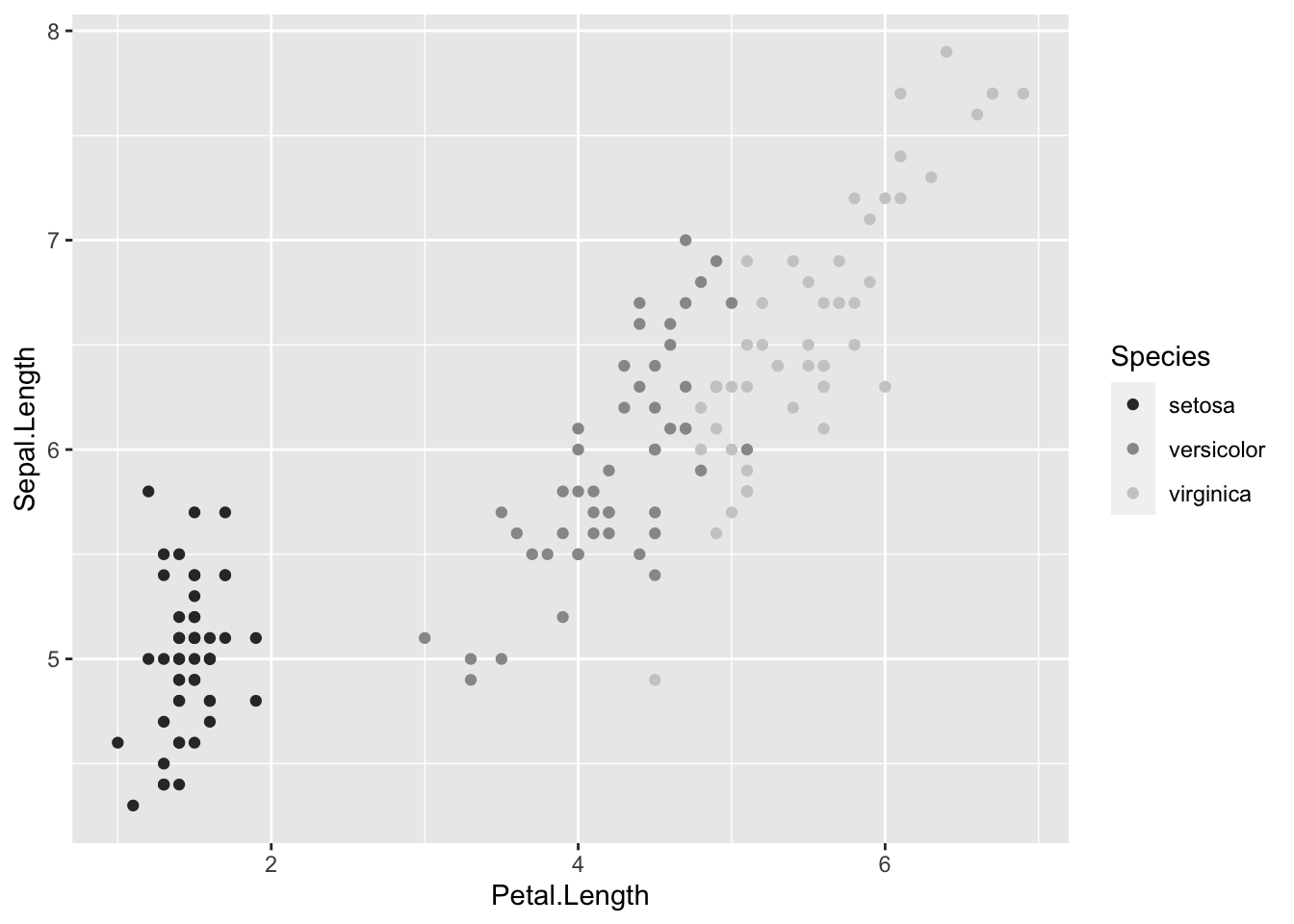print(IrisBox + scale_fill_grey())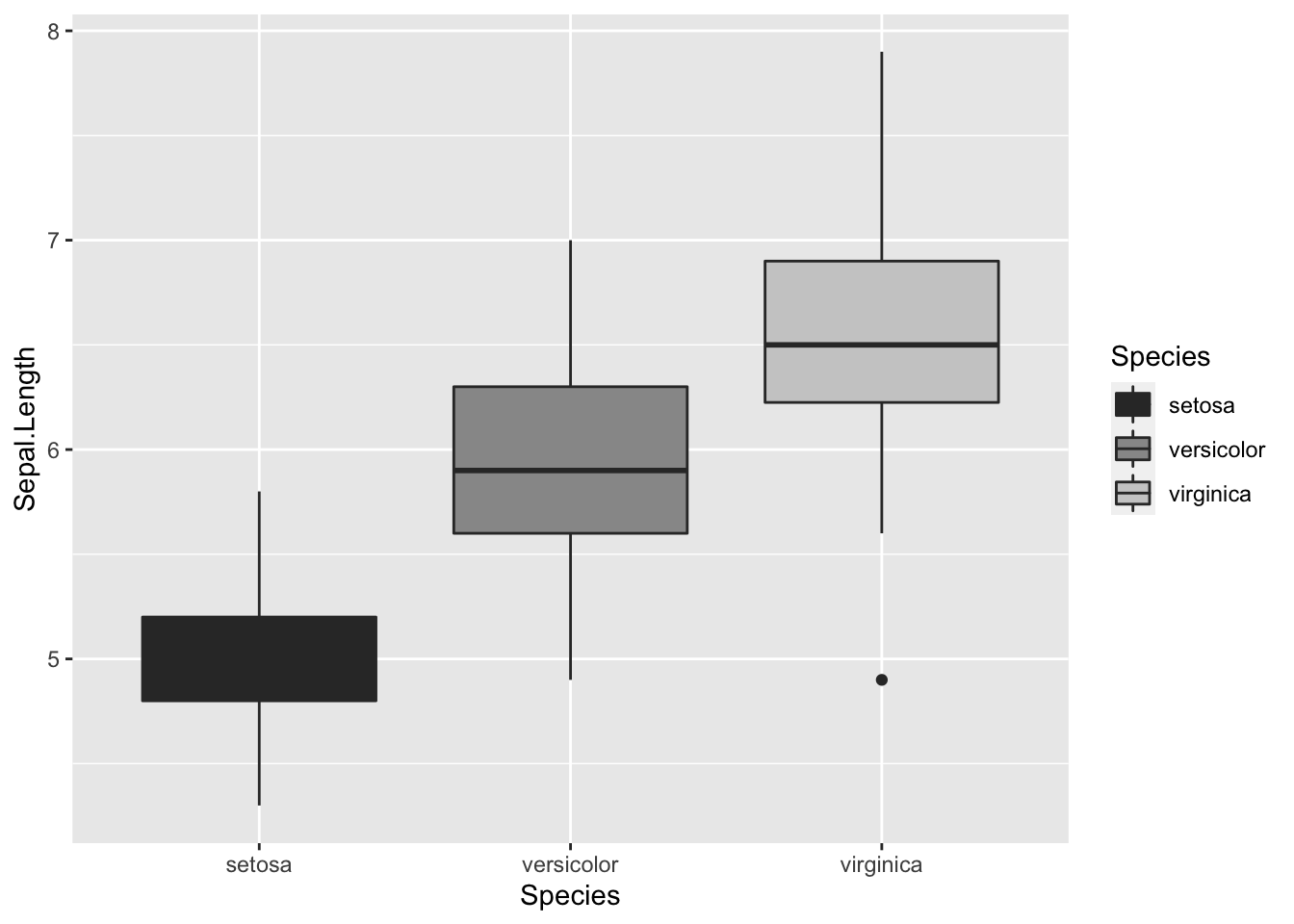Assign colours from a pre-made pallette. Use + scale_colour_brewer() or + scale_fill_brewer. To do this you will need to install the package RColorBrewer and load in R.

library(RColorBrewer)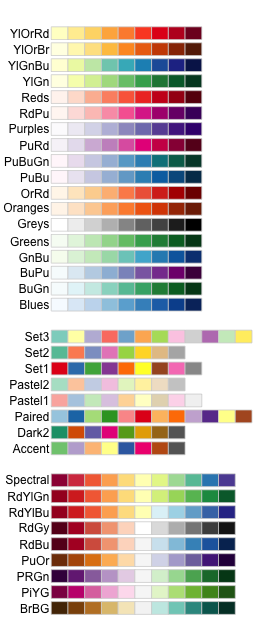This can then be added to the end of your graph code just like the others + scale_colour_brewer(palette = "chosen.palette") for scatterplots and + scale_fill_brewer(palette = "chosen.palette") for boxplots, where "chosen.pallete" is one of the available palletes. For example,

print(IrisPlot + scale_colour_brewer(palette = "Dark2"))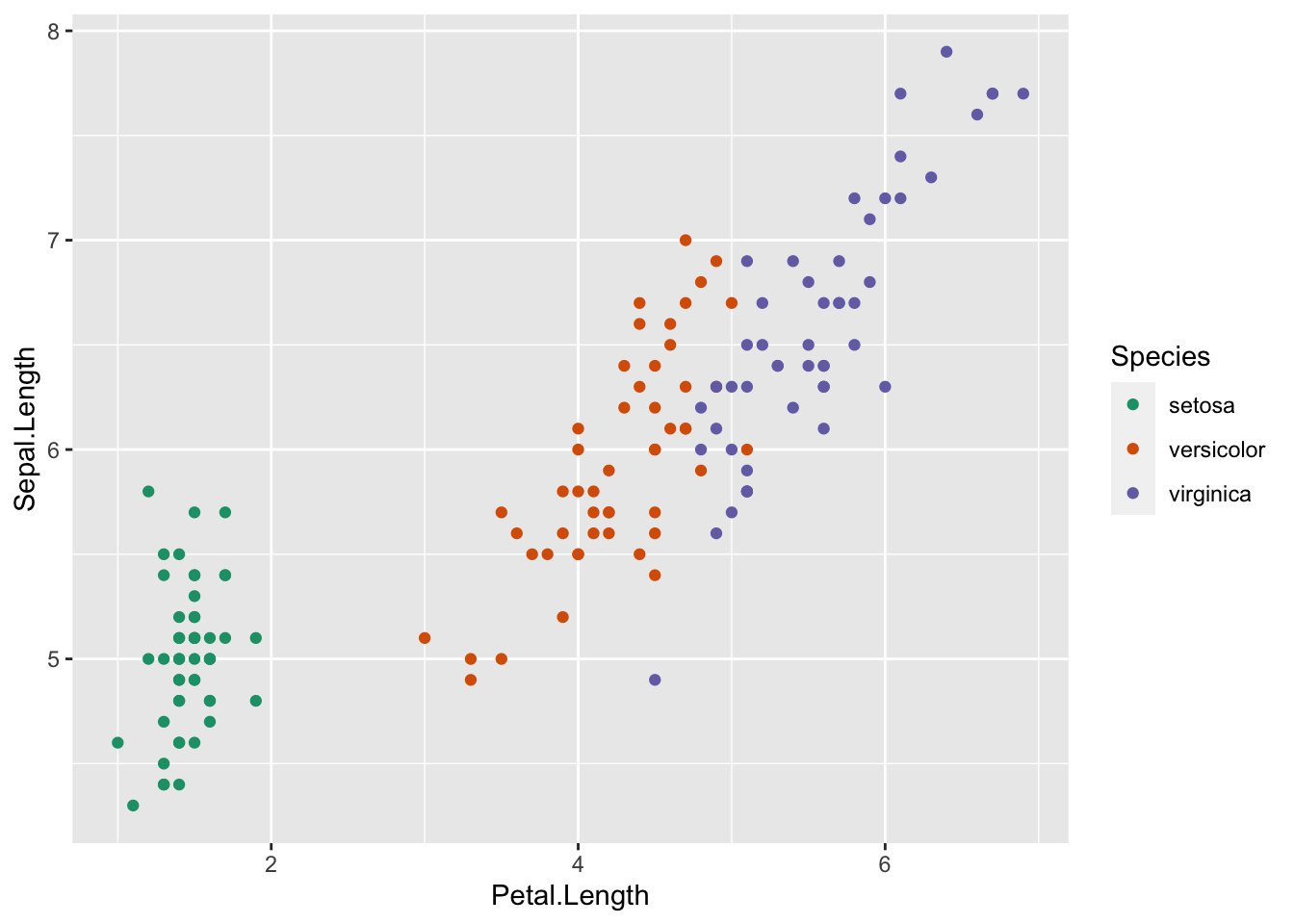print(IrisBox + scale_fill_brewer(palette = "Oranges"))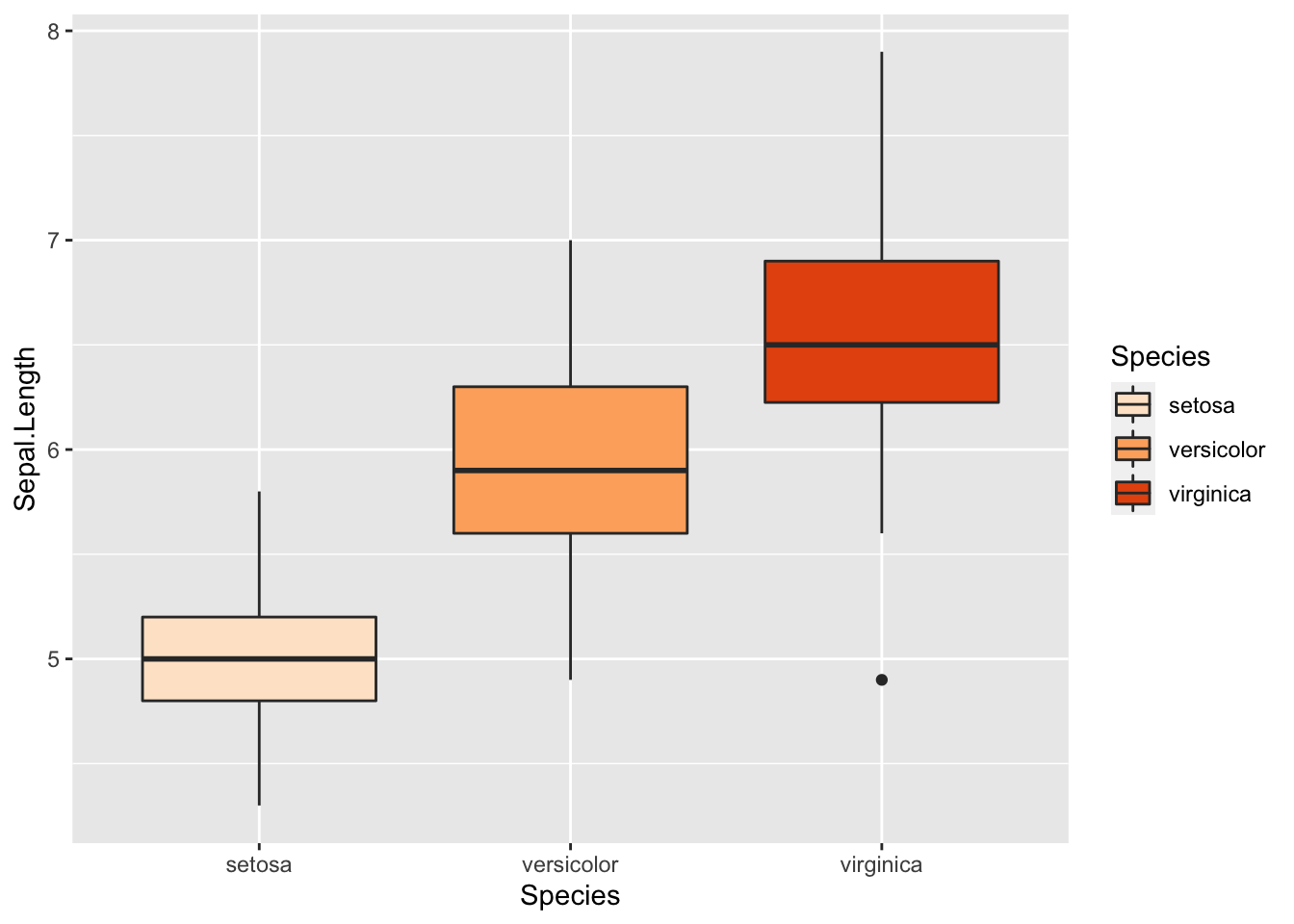### Changing symbols in a scatterplot

In a simple scatterplot with no grouping variables, you can change the shape of the symbol by adding shape = ? to the geom_point() code, where ? is one of the following numbers for different shapes.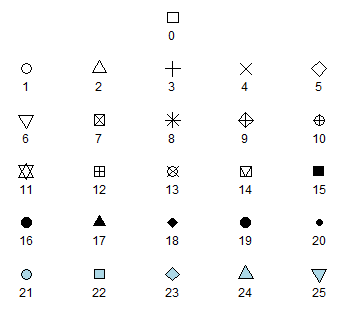For example, to use a filled triangle,

IrisPlot <- ggplot(iris, aes(Petal.Length, Sepal.Length)) +
geom_point(shape = 17)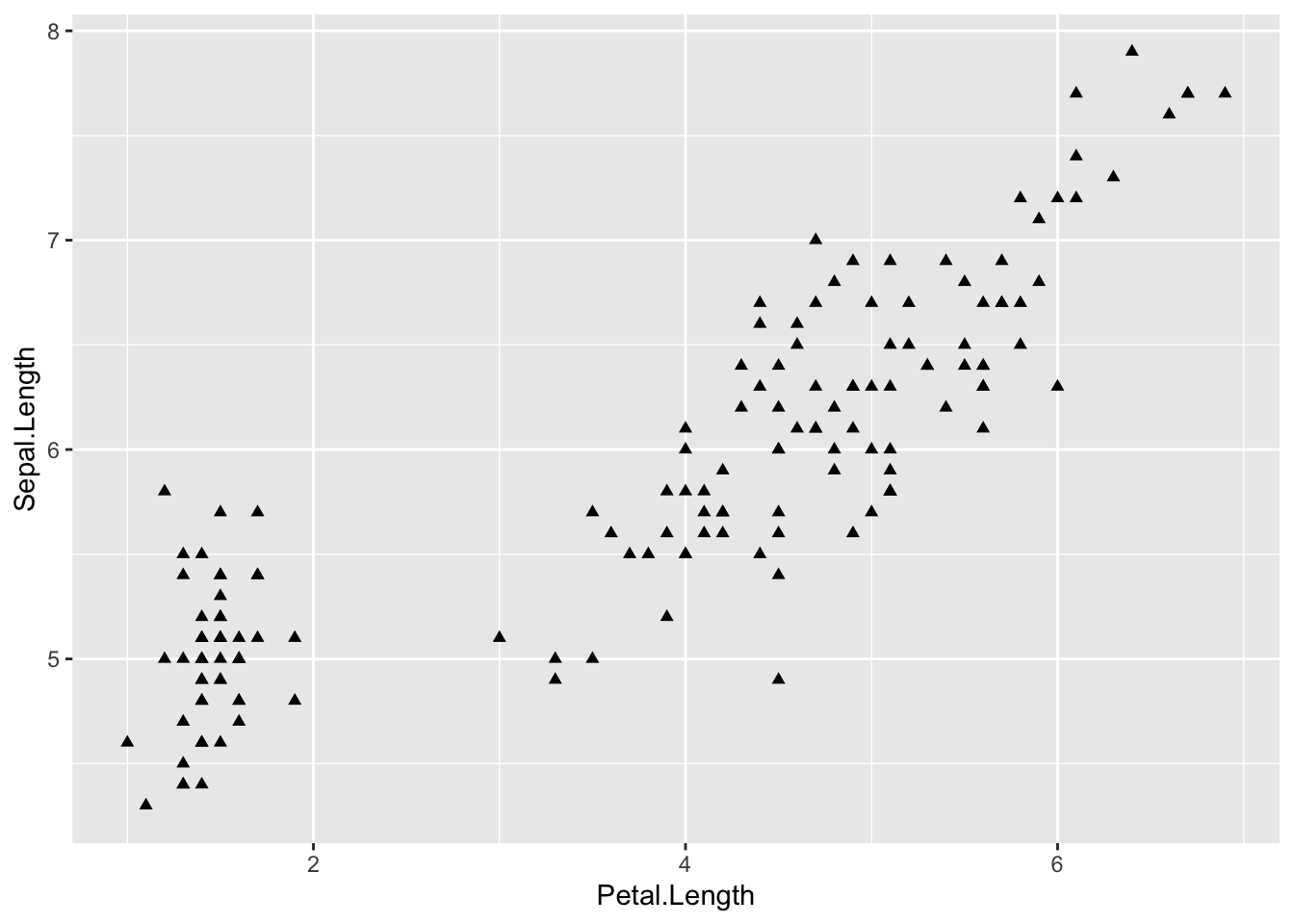Different symbols can be used to group data in a scatterplot. This can be very helpful when printing in black and white or to further distinguish your categories.

To do this, you need to add shape = variable.name within your basic plot aes brackets, where variable.name is the name of your grouping variable. For example, to have different symbols for each species, we would use.

IrisPlot.shape <- ggplot(iris, aes(Petal.Length, Sepal.Length, shape = Species)) +
geom_point()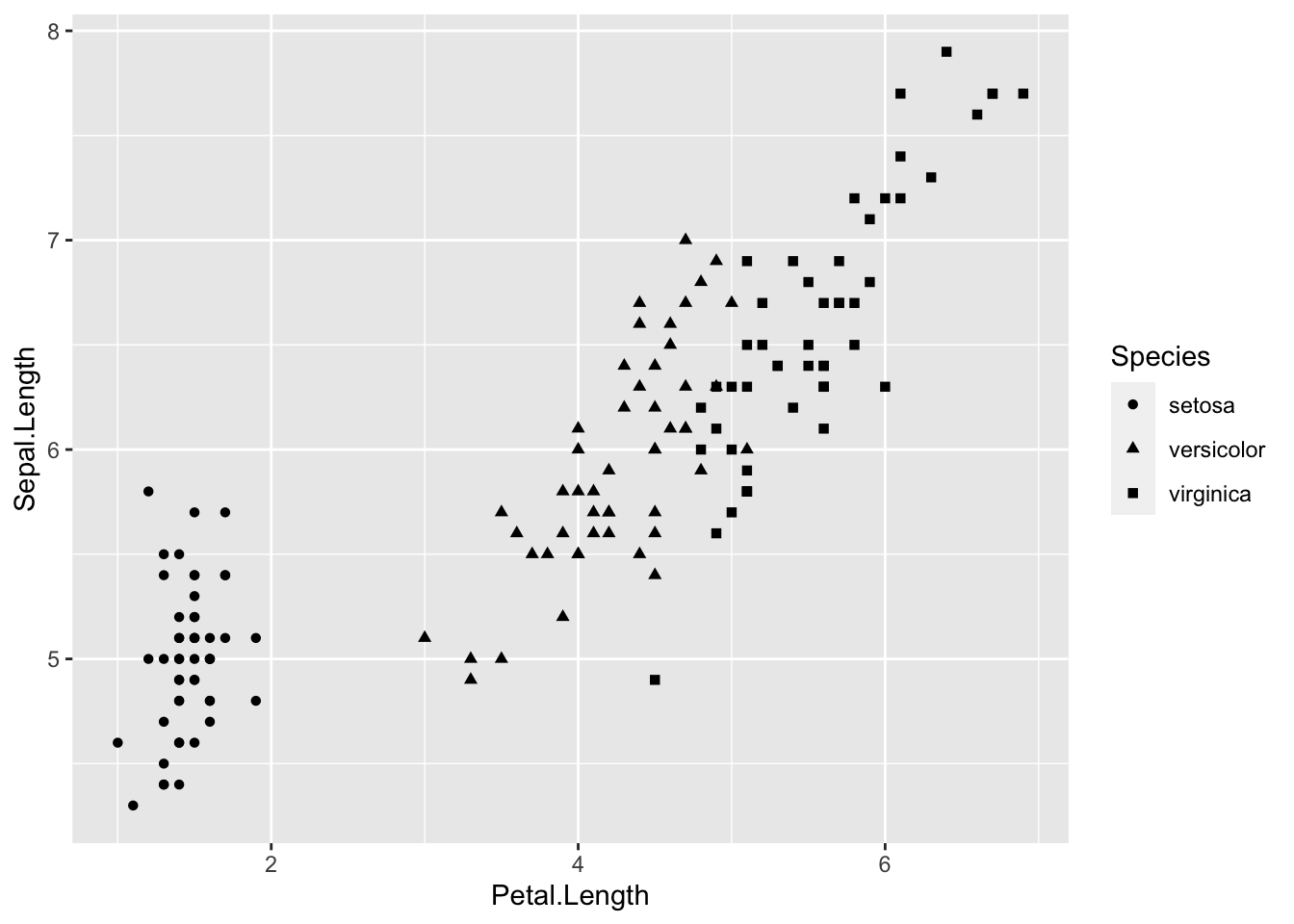To set the symbols manually, we can use the symbol codes in scale_shape_manual() added to your print function.

print(IrisPlot.shape + scale_shape_manual(values = c(0, 16, 3)))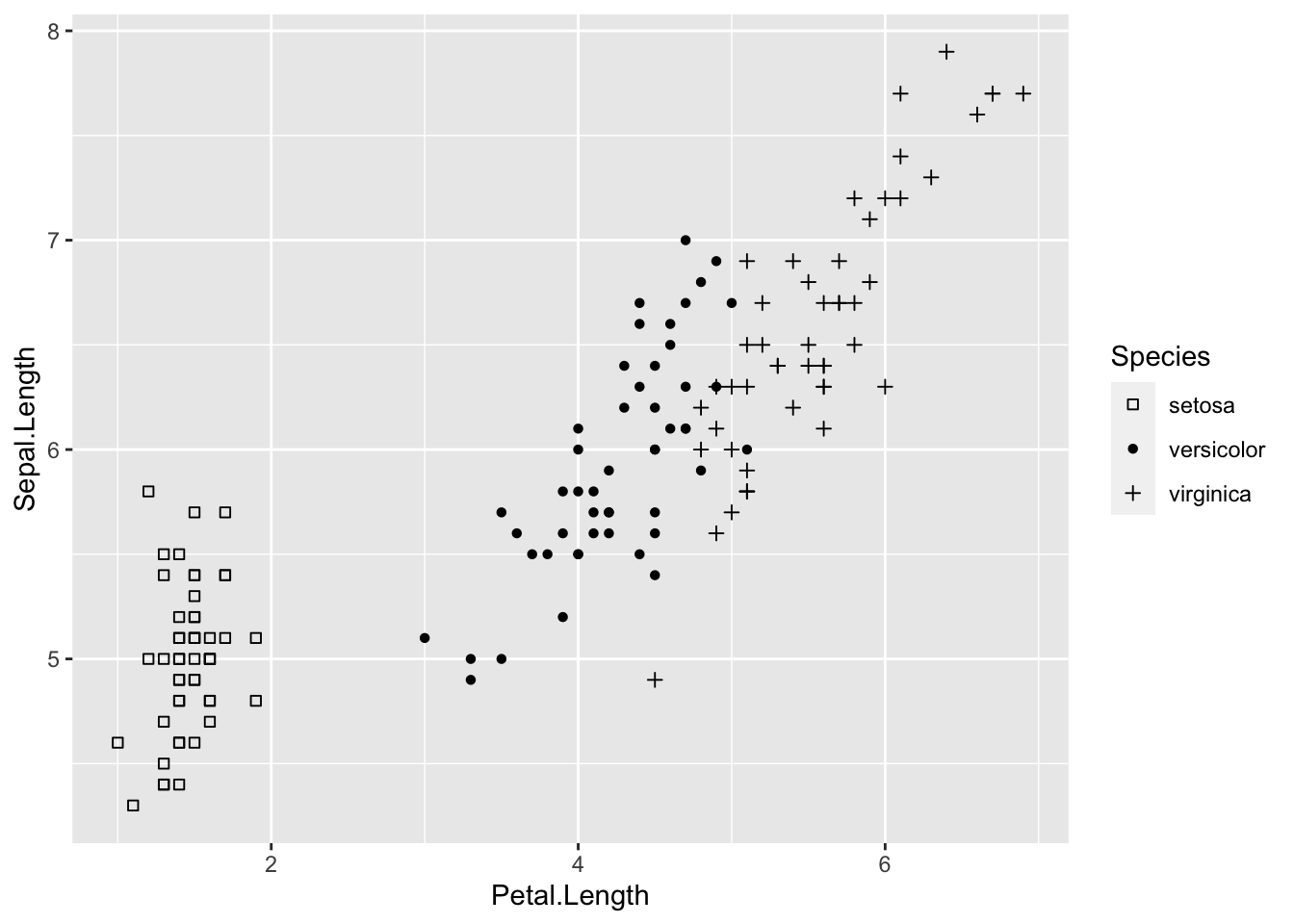This can be used with colour to further distinguish and group your variables.

IrisPlot.shape <- ggplot(iris, aes(Petal.Length, Sepal.Length, shape = Species, colour = Species)) +
geom_point()

print(IrisPlot.shape + scale_shape_manual(values = c(0, 16, 3)) + scale_colour_manual(values = c("chartreuse4", "chocolate", "slateblue4")))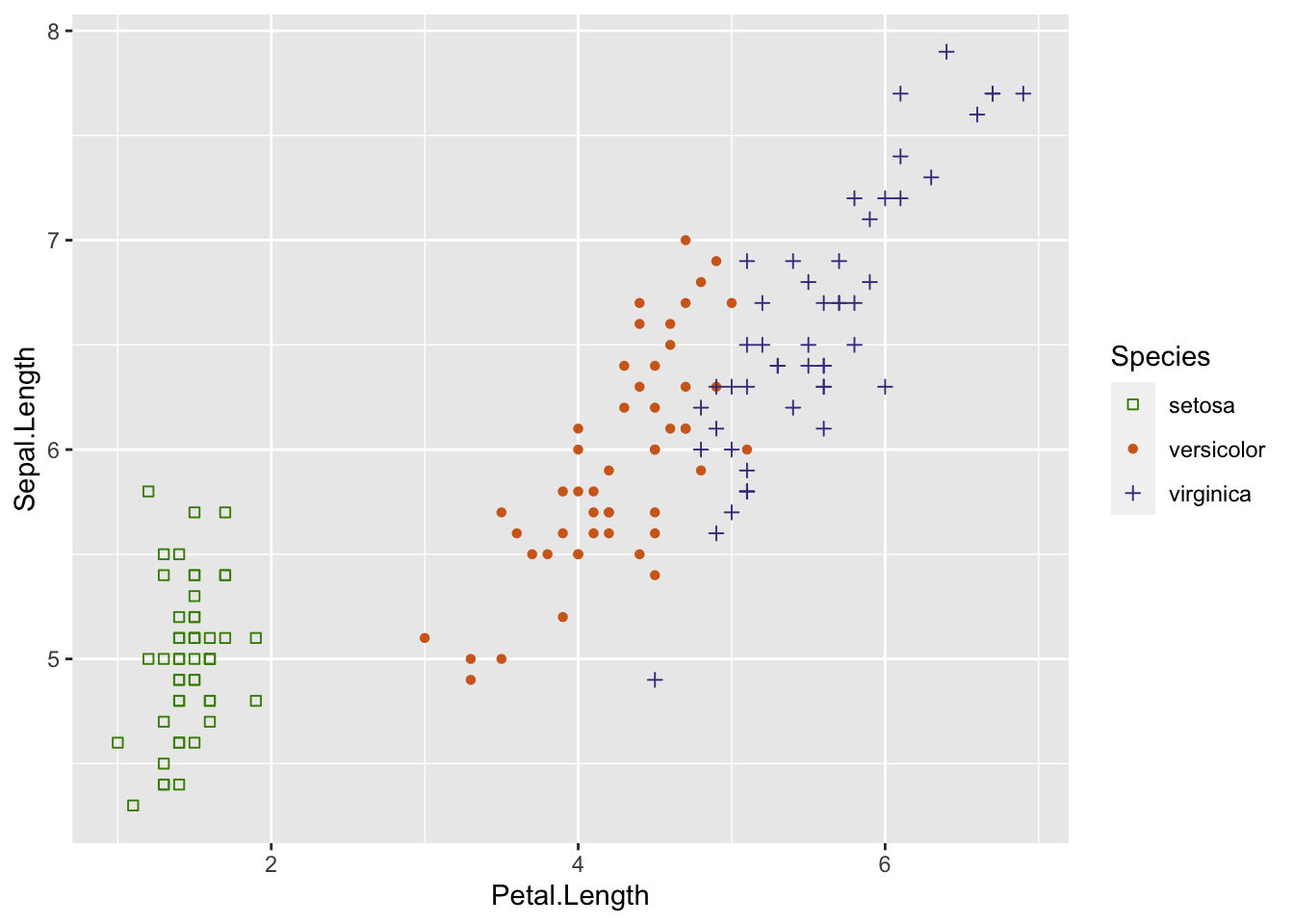###Further help

To further customise the aesthetics of the graph, including colour and formatting, see our other ggplot help pages:
altering overall appearance.